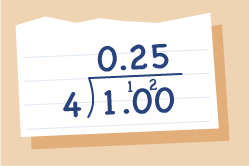Everyday maths 2 (Wales)

Start this free course now. Just create an account and sign in. Enrol and complete the course for a free statement of participation or digital badge if available.

# 9.3 Changing a fraction to a decimal

Again there are two ways to do this, both based on the two methods just shown for changing a fraction to a percentage.

## Method 1

### Example: Change the fraction into a percentage and divide by 100

× = which cancels to = 25%

Now convert to a decimal by dividing by 100:

• 25 ÷ 100 = 0.25

## Method 2

### Example: Divide the top of the fraction by the bottom

= 1 ÷ 4 = 0.25.Figure 13 Expressed as a decimal: 1 ÷ 4

## Activity 23: Changing a fraction to a decimal

Express these fractions as decimals:

1.

2.

1. 0.4

2. 0.125

3. 0.3

Fractions and percentages deal with splitting numbers into a given number of equal portions, or parts. When dealing with the next topic, ratio, you will still be splitting quantities into a given number of parts, but when sharing in a ratio, you do not share evenly. This might sound a little complicated but you’ll have been doing it since you were a child.

## Summary

In this section you have:

• learned about the relationship between fractions, decimals and percentages and are now able to convert between the three.#### Find out more

*Eligibility rules apply for financial support.